# Simplify Expressions with Exponents

Simplify a variety of algebraic expressions using the laws of exponents:

## Example 1

Simplify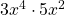It is important to remember that the exponent applies to only what it is written beside. The exponentapplies to the, not to the. Written out in full we have:In short,## Example 2

Simplify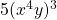The exponent 3 is applied to each factor in the bracket: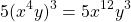## Example 3

SimplifyA negative exponent means to divide repeatedly. We ‘take the reciprocal‘. The result here is a positive number.## Example 4

SimplifyLet’s see what the result is of a negative exponent on the denominator in general:

SimplifyIn short,Applying this new law, we haveand.## Example 5

SimplifyIt is helpful to gather like factors as follows: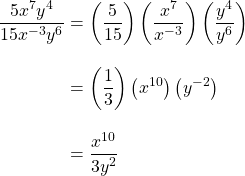## Example 6

SimplifyLet’s make another new law.

SimplifyIn short,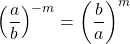Apply this new law to example 6 we haveWorksheet